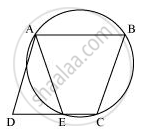# ABCD is a parallelogram. The circle through A, B and C intersect CD (produced if necessary) at E. Prove that AE = AD. - Mathematics

ABCD is a parallelogram. The circle through A, B and C intersect CD (produced if necessary) at E. Prove that AE = AD.

#### SolutionIt can be observed that ABCE is a cyclic quadrilateral and in a cyclic quadrilateral, the sum of the opposite angles is 180°.

∠AEC + ∠CBA = 180°

∠AEC + ∠AED = 180° (Linear pair)

∠AED = ∠CBA ... (1)

For a parallelogram, opposite angles are equal.

From (1) and (2),

AD = AE (Angles opposite to equal sides of a triangle)

Is there an error in this question or solution?
Chapter 10: Circles - Exercise 10.6 [Page 186]

#### APPEARS IN

NCERT Class 9 Maths
Chapter 10 Circles
Exercise 10.6 | Q 6 | Page 186

Share